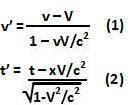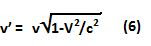## Tuesday, December 29, 2009

### Irodov Problem 1.365

a) Let the direction of motion of the frame K' be assigned as the x-axis. Let v', x' and t' be the instantaneous velocity, position and time of the moving body as seen by the observer in frame K'. Since, the direction of motion of the accelerating body coincides with the motion of the frame K' , x -axis and x' axis coincide. From the relativistic transformations for velocity and time we have,The acceleration of the body as seen from frame K' is then given by,(b) In this problem the body moves along the y-axis while the frame K' moves along the x-axis. Thus, using relativistic transformations we have,The acceleration of the body as seen in frame K' is then given by,